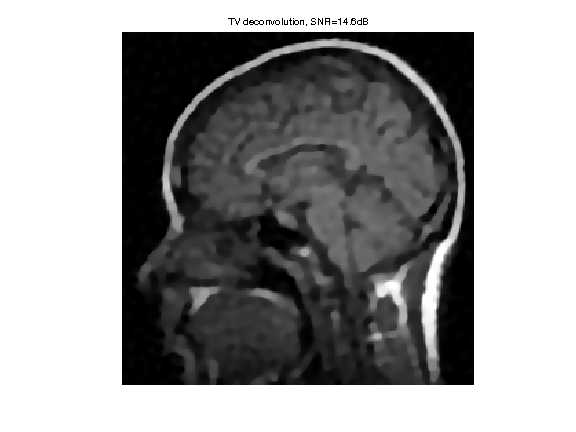# Image Deconvolution using Sparse Regularization¶

Important: Please read the installation page for details about how to install the toolboxes. $\newcommand{\dotp}{\langle #1, #2 \rangle}$ $\newcommand{\enscond}{\lbrace #1, #2 \rbrace}$ $\newcommand{\pd}{ \frac{ \partial #1}{\partial #2} }$ $\newcommand{\umin}{\underset{#1}{\min}\;}$ $\newcommand{\umax}{\underset{#1}{\max}\;}$ $\newcommand{\umin}{\underset{#1}{\min}\;}$ $\newcommand{\uargmin}{\underset{#1}{argmin}\;}$ $\newcommand{\norm}{\|#1\|}$ $\newcommand{\abs}{\left|#1\right|}$ $\newcommand{\choice}{ \left\{ \begin{array}{l} #1 \end{array} \right. }$ $\newcommand{\pa}{\left(#1\right)}$ $\newcommand{\diag}{{diag}\left( #1 \right)}$ $\newcommand{\qandq}{\quad\text{and}\quad}$ $\newcommand{\qwhereq}{\quad\text{where}\quad}$ $\newcommand{\qifq}{ \quad \text{if} \quad }$ $\newcommand{\qarrq}{ \quad \Longrightarrow \quad }$ $\newcommand{\ZZ}{\mathbb{Z}}$ $\newcommand{\CC}{\mathbb{C}}$ $\newcommand{\RR}{\mathbb{R}}$ $\newcommand{\EE}{\mathbb{E}}$ $\newcommand{\Zz}{\mathcal{Z}}$ $\newcommand{\Ww}{\mathcal{W}}$ $\newcommand{\Vv}{\mathcal{V}}$ $\newcommand{\Nn}{\mathcal{N}}$ $\newcommand{\NN}{\mathcal{N}}$ $\newcommand{\Hh}{\mathcal{H}}$ $\newcommand{\Bb}{\mathcal{B}}$ $\newcommand{\Ee}{\mathcal{E}}$ $\newcommand{\Cc}{\mathcal{C}}$ $\newcommand{\Gg}{\mathcal{G}}$ $\newcommand{\Ss}{\mathcal{S}}$ $\newcommand{\Pp}{\mathcal{P}}$ $\newcommand{\Ff}{\mathcal{F}}$ $\newcommand{\Xx}{\mathcal{X}}$ $\newcommand{\Mm}{\mathcal{M}}$ $\newcommand{\Ii}{\mathcal{I}}$ $\newcommand{\Dd}{\mathcal{D}}$ $\newcommand{\Ll}{\mathcal{L}}$ $\newcommand{\Tt}{\mathcal{T}}$ $\newcommand{\si}{\sigma}$ $\newcommand{\al}{\alpha}$ $\newcommand{\la}{\lambda}$ $\newcommand{\ga}{\gamma}$ $\newcommand{\Ga}{\Gamma}$ $\newcommand{\La}{\Lambda}$ $\newcommand{\si}{\sigma}$ $\newcommand{\Si}{\Sigma}$ $\newcommand{\be}{\beta}$ $\newcommand{\de}{\delta}$ $\newcommand{\De}{\Delta}$ $\newcommand{\phi}{\varphi}$ $\newcommand{\th}{\theta}$ $\newcommand{\om}{\omega}$ $\newcommand{\Om}{\Omega}$

This numerical tour explores the use of sparsity regularization to perform image deconvolution.

In :
addpath('toolbox_signal')


## Sparse Regularization¶

This tour consider measurements $y=\Phi f_0 + w$ where $\Phi$ is a convolution $\Phi f = h \star f$ and $w$ is an additive noise.

This tour is focussed on using sparsity to recover an image from the measurements $y$. It consider a synthesis-based regularization, that compute a sparse set of coefficients $(a_m^{\star})_m$ in a frame $\Psi = (\psi_m)_m$ that solves $$a^{\star} \in \text{argmin}_a \: \frac{1}{2}\|y-\Phi \Psi a\|^2 + \lambda J(a)$$

where $\lambda$ should be adapted to the noise level $\|w\|$

Here we used the notation $$\Psi a = \sum_m a_m \psi_m$$ to indicate the reconstruction operator, and $J(a)$ is the $\ell^1$ sparsity prior $$J(a)=\sum_m \|a_m\|.$$

## Image Blurring¶

Deconvolution corresponds to removing a blur from an image. We use here a Gaussian blur.

Parameters for the tour: width of the kernel (in pixel) and noise level.

In :
setting = 1;
switch setting
case 1
% difficult
s = 3;
sigma = .02;
case 2
% easy
s = 1.2;
sigma = .02;
end


First we load the image to be processed.

In :
n = 128*2;
name = 'lena';
name = 'boat';
name = 'mri';
f0 = rescale(crop(f0,n));


Display it.

In :
clf;
imageplot(f0);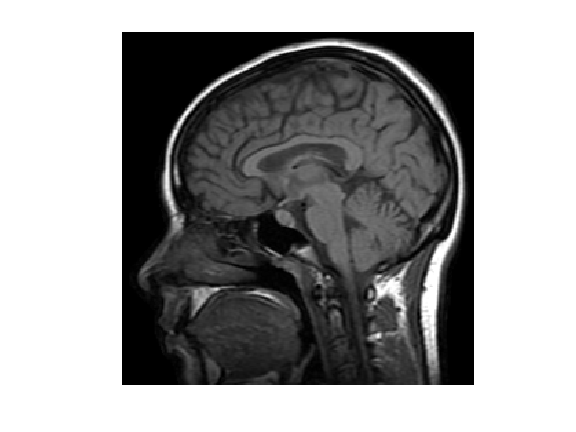We build a convolution kernel. Since we are going to use Fourier to compute the convolution, we set the center of the kernel in the (1,1) pixel location.

Kernel.

In :
x = [0:n/2-1, -n/2:-1];
[Y,X] = meshgrid(x,x);
h = exp( (-X.^2-Y.^2)/(2*s^2) );
h = h/sum(h(:));


Useful for later : the Fourier transform (should be real because of symmetry).

In :
hF = real(fft2(h));


Display the kernel $h$ and its transform $\hat h$. We use |fftshift| to center the filter for display.

In :
clf;
imageplot(fftshift(h), 'Filter', 1,2,1);
imageplot(fftshift(hF), 'Fourier transform', 1,2,2);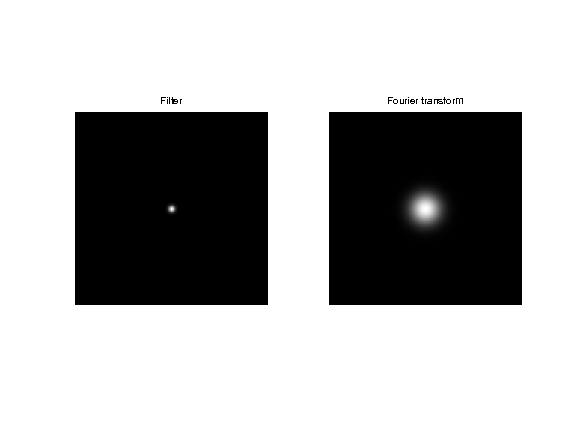We use this short hand for the filtering. Scilab user should define a function in a separate file to perform this. Note that this is a symmetric operator.

In :
Phi = @(x)real(ifft2(fft2(x).*hF));


Apply the filter.

In :
y0 = Phi(f0);


Display the filtered observation.

In :
clf;
imageplot(f0, 'Image f0', 1,2,1);
imageplot(y0, 'Observation without noise', 1,2,2);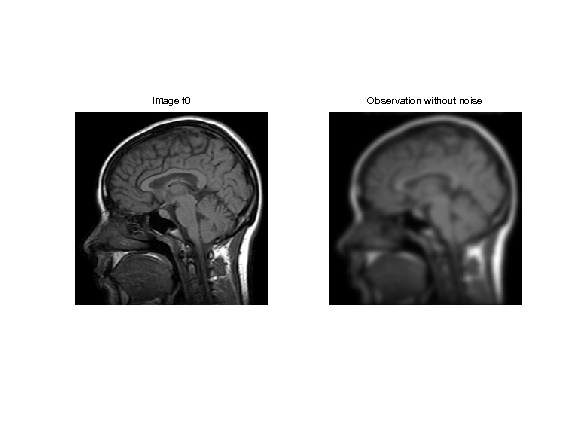Add some noise of variance $\sigma^2$, to obtain $y=\Phi f_0 + w = f_0 \star h + w$.

In :
y = y0 + randn(n,n)*sigma;


Display.

In :
clf;
imageplot(y0, 'Observation without noise', 1,2,1);
imageplot(clamp(y), 'Observation with noise', 1,2,2);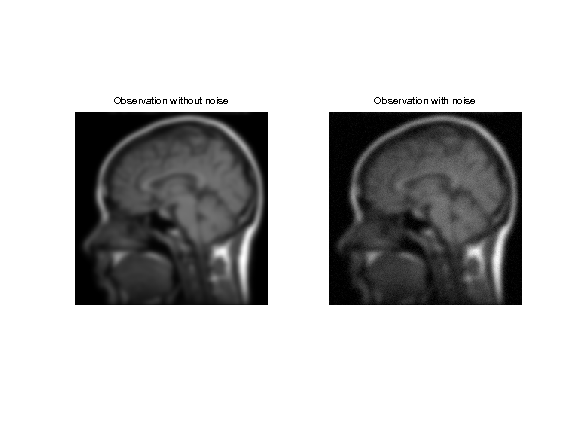## Soft Thresholding in a Basis¶

The soft thresholding operator is at the heart of $\ell^1$ minimization schemes. It can be applied to coefficients $a$, or to an image $f$ in an ortho-basis.

The soft thresholding is a 1-D functional that shrinks the value of coefficients. $$s_T(u)=\max(0,1-T/|u|)u$$

Define a shortcut for this soft thresholding 1-D functional.

In :
SoftThresh = @(x,T)x.*max( 0, 1-T./max(abs(x),1e-10) );


Display a curve of the 1D soft thresholding.

In :
clf;
T = linspace(-1,1,1000);
plot( T, SoftThresh(T,.5) );
axis('equal');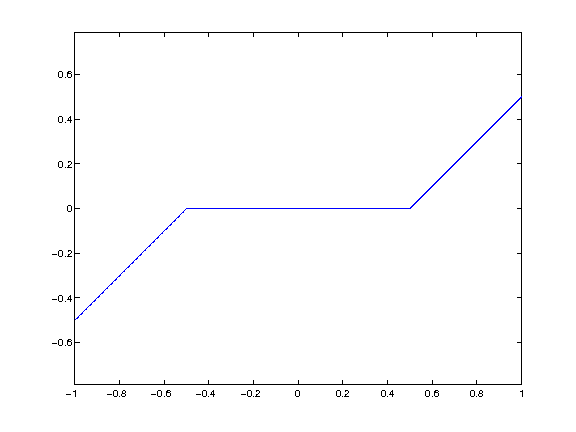Note that the function |SoftThresh| can also be applied to vector (because of Matlab/Scilab vectorialized computation), which defines an operator on coefficients: $$S_T(a) = ( s_T(a_m) )_m.$$

In the next section, we use an orthogonal wavelet basis $\Psi$.

We set the parameters of the wavelet transform.

In :
Jmax = log2(n)-1;
Jmin = Jmax-3;


Shortcut for $\Psi$ and $\Psi^*$ in the orthogonal case.

In :
options.ti = 0; % use orthogonality.
Psi = @(a)perform_wavelet_transf(a, Jmin, -1,options);
PsiS = @(f)perform_wavelet_transf(f, Jmin, +1,options);


The soft thresholding opterator in the basis $\Psi$ is defined as $$S_T^\Psi(f) = \sum_m s_T( \langle f,\psi_m \rangle ) \psi_m$$

It thus corresponds to applying the transform $\Psi^*$, thresholding the coefficients using $S_T$ and then undoing the transform using $\Psi$. $$S_T^\Psi(f) = \Psi \circ S_T \circ \Psi^*$$

In :
SoftThreshPsi = @(f,T)Psi(SoftThresh(PsiS(f),T));


This soft thresholding corresponds to a denoising operator.

In :
clf;
imageplot( clamp(SoftThreshPsi(f0,.1)) );## Deconvolution using Orthogonal Wavelet Sparsity¶

If $\Psi$ is an orthogonal basis, a change of variable shows that the synthesis prior is also an analysis prior, that reads $$f^{\star} \in \text{argmin}_f \: E(f) = \frac{1}{2}\|y-\Phi f\|^2 + \lambda \sum_m \|\langle f,\psi_m \rangle\|.$$

To solve this non-smooth optimization problem, one can use forward-backward splitting, also known as iterative soft thresholding.

It computes a series of images $f^{(\ell)}$ defined as $$f^{(\ell+1)} = S_{\tau\lambda}^{\Psi}( f^{(\ell)} - \tau \Phi^{*} (\Phi f^{(\ell)} - y) )$$

Set up the value of the threshold.

In :
lambda = .02;


In our setting, since $h$ is symmetric, one has $\Phi^* f = \Phi f = f \star h$.

For $f^{(\ell)}$ to converge to a solution of the problem, the gradient step size should be chosen as $$\tau < \frac{2}{\|\Phi^* \Phi\|}$$

Since the filtering is an operator of norm 1, this must be smaller than 2.

In :
tau = 1.5;


Number of iterations.

In :
niter = 100;


Initialize the solution.

In :
fSpars = y;


First step: perform one step of gradient descent of the energy $\|y-f\star h\|^2$.

In :
fSpars = fSpars + tau * Phi( y-Phi(fSpars) );


Second step: denoise the solution by thresholding.

In :
fSpars = SoftThreshPsi( fSpars, lambda*tau );


Exercise 1

Perform the iterative soft thresholding. Monitor the decay of the energy $E$ you are minimizing.

In :
exo1()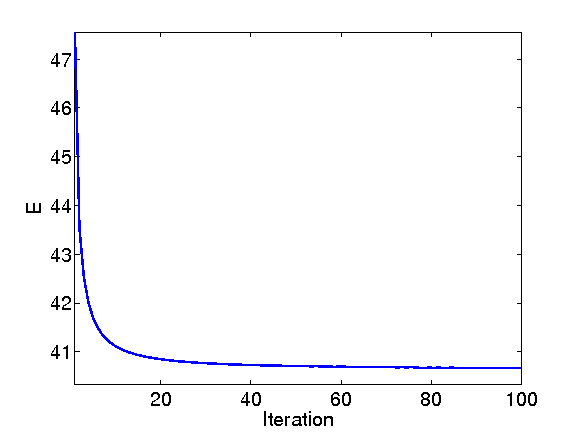In :
%% Insert your code here.


Display the result.

In :
clf;
imageplot(clamp(fSpars), ['Sparsity deconvolution, SNR=' num2str(snr(f0,fSpars),3) 'dB']);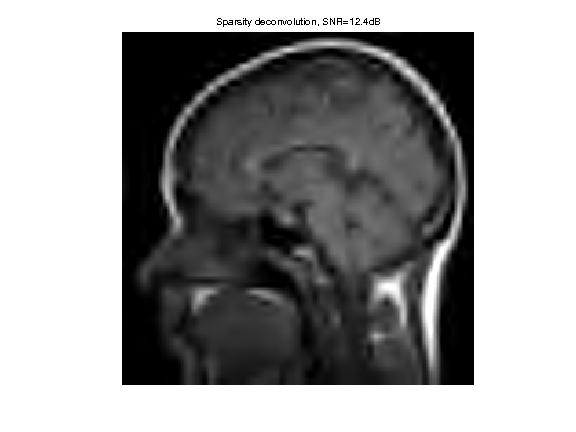Exercise 2

Try to find the best threshold $\lambda$. To this end, perform a lot of iterations, and progressively decay the threshold $\lambda$ during the iterations. Record the best result in |fBestOrtho|. armup escent

In :
exo2()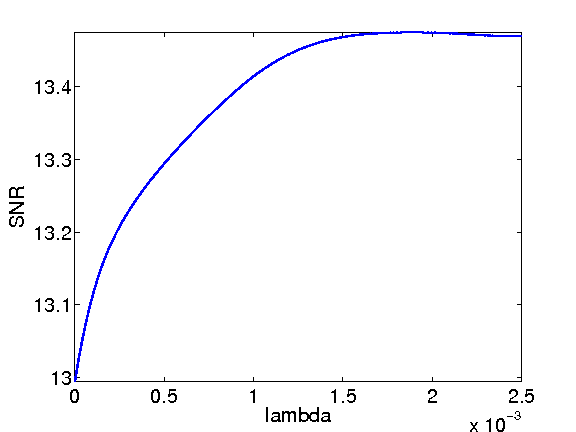In :
%% Insert your code here.


Display the result.

In :
clf;
imageplot(clamp(fBestOrtho), ['Sparsity deconvolution, SNR=' num2str(snr(f0,fBestOrtho),3) 'dB']);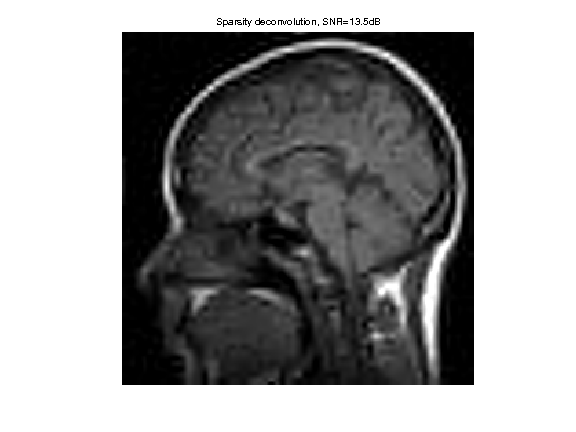## Deconvolution using Translation Invariant Wavelet Sparsity¶

Orthogonal sparsity performs a poor regularization because of the lack of translation invariance. This regularization is enhanced by considering $\Psi$ as a redundant tight frame of translation invariant wavelets.

One thus looks for optimal coefficients $a^\star$ that solves $$a^{\star} \in \text{argmin}_a \: \frac{1}{2}\|y-\Phi \Psi a\|^2 + \lambda J(a)$$

One should be careful that because of the redundancy of the wavelet tight frame, one should use a weighted $\ell^1$ norm, where each coefficient is divided by the number of redundancy at each scale. $$J(a) = \sum_{j,k} u_j \|a_{j,k}\|$$

where the wavelet coefficients $a_{m} = a_{j,k}$ are indexed by the scale (and orientation) $j$ and the location $k$.

Compute the scaling factor (inverse of the redundancy).

In :
J = Jmax-Jmin+1;
u = [4^(-J) 4.^(-floor(J+2/3:-1/3:1)) ];


Value of the regularization parameter.

In :
lambda = .01;


Shortcut for the wavelet transform. Important: Note that |PsiS| is the shortcut for $\Psi^*$, but |Psi| is the shortcut for $\Xi = (\Psi^*)^+$ that satisfy $\Xi \Phi^* f = f$.

In :
options.ti = 1; % use translation invariance
Psi = @(a)perform_wavelet_transf(a, Jmin, -1,options);
PsiS = @(f)perform_wavelet_transf(f, Jmin, +1,options);


The forward-backward algorithm now compute a series of wavelet coefficients $a^{(\ell)}$ computed as $$a^{(\ell+1)} = S_{\tau\lambda}( a^{(\ell)} + \Psi^*\Phi( y - \Phi\Xi a^{(\ell)} ) ).$$

The soft thresholding is defined as: $$\forall m, \quad S_T(a)_m = \max(0, 1-T/\|a_m\|)a_m.$$

The step size should satisfy: $$\tau < \frac{2}{\|\Phi\Psi\|^2}.$$

In :
tau = 1.5;


Initialize the wavelet coefficients with those of the observations.

In :
a = PsiS(y);


In :
a = a + tau * PsiS( Phi( y-Phi(Psi(a)) ) );


Soft threshold.

In :
a = SoftThresh( a, lambda*tau );


Important: keep in mind that the prior $J(a)$ is a weighted $\ell^1$ norm, it should thus be computed this way:

In :
U = repmat( reshape(u,[1 1 length(u)]), [n n 1] );
Ja = sum(sum(sum( abs(a.*U) )));


Exercise 3

Perform the iterative soft thresholding. Monitor the decay of the energy.

In :
exo3()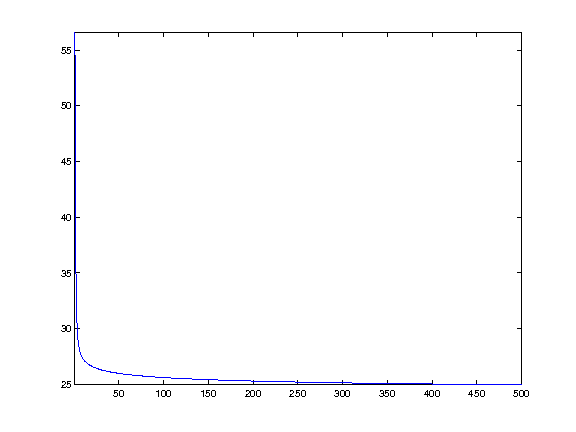In :
%% Insert your code here.


Perform the reconstruction.

In :
fTI = Psi(a);


Display the result.

In :
clf;
imageplot(fTI);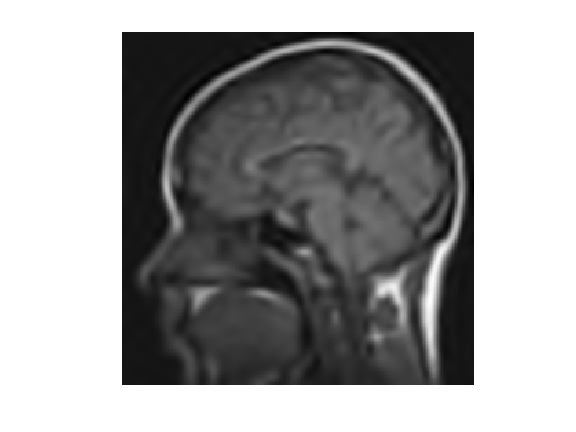Exercise 4

Compute the optimal value of $\lambda$, and record the optimal reconstruction |fBestTI|. armup escent

In :
exo4()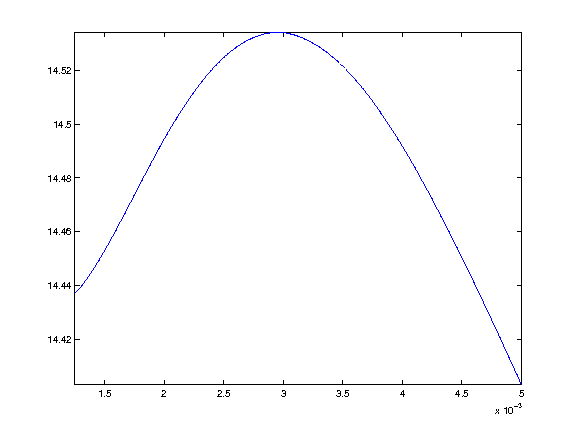In :
%% Insert your code here.


Display the result.

In :
clf;
imageplot(clamp(fBestTI), ['Sparsity deconvolution TI, SNR=' num2str(snr(f0,fBestTI),3) 'dB']);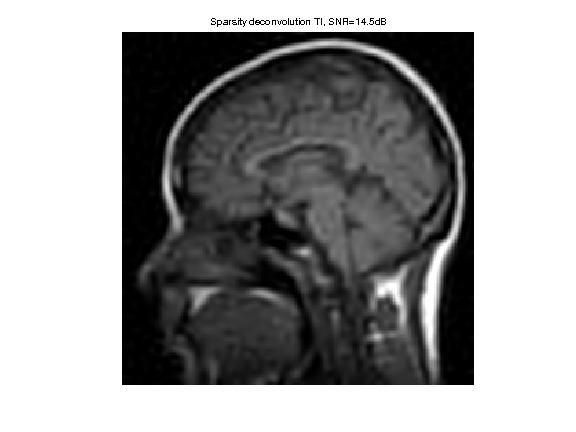Exercise 5

Compare with the result of TV regularization, record the optimal TV result in |fBestTV|.

In :
exo5()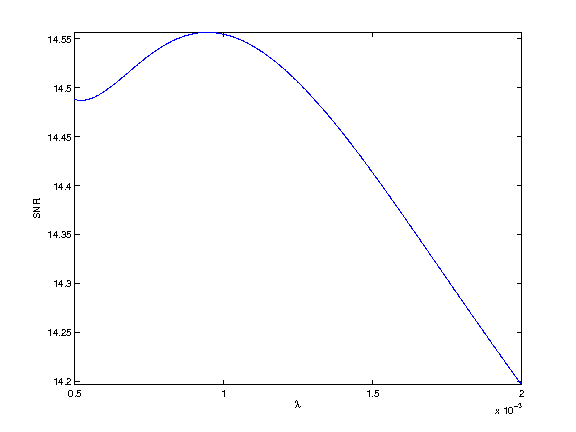In :
%% Insert your code here.


Display the result.

In :
clf;
imageplot(clamp(fBestTV), ['TV deconvolution, SNR=' num2str(snr(f0,fBestTV),3) 'dB']);